# 如何在Tableau中更好的呈现和计算工作日收入

### 介绍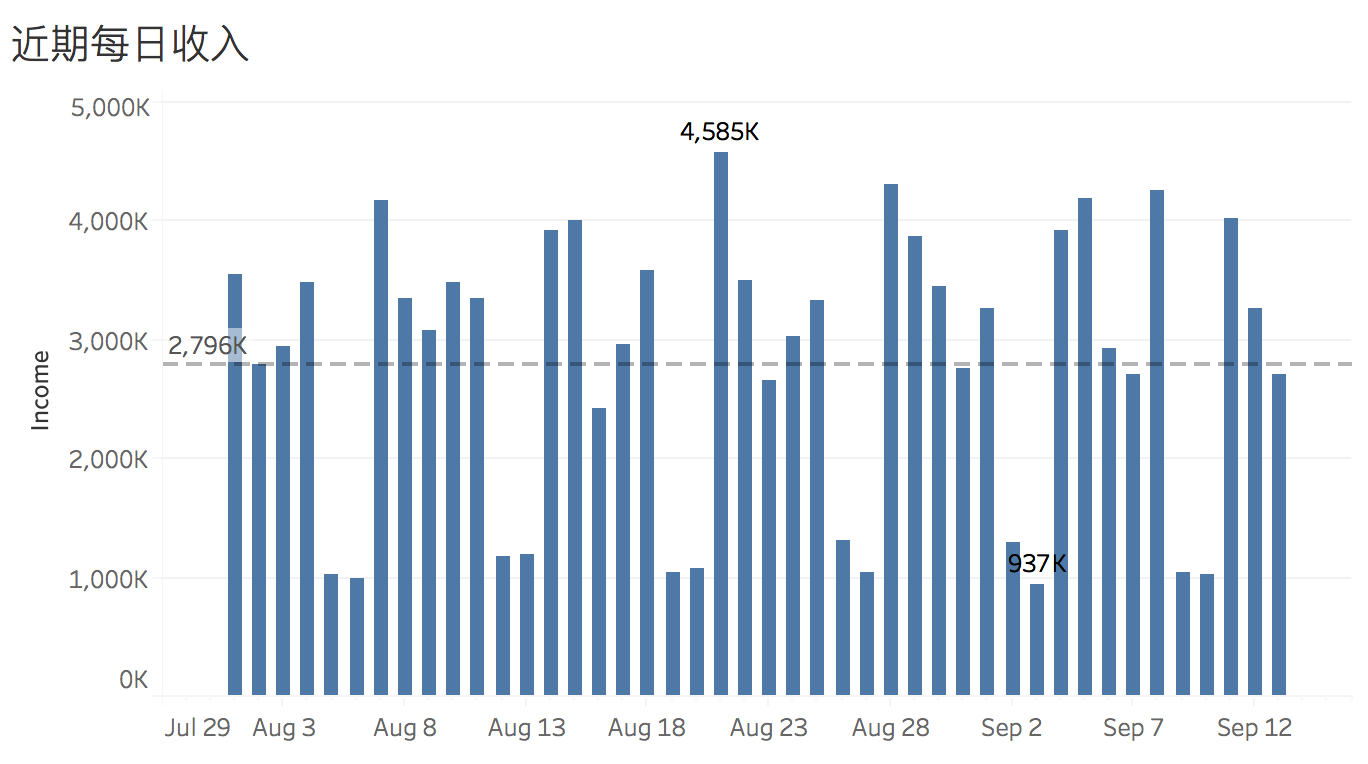``````字段名：is_weekend
DATEPART('weekday', [Date]) = 1 or DATEPART('weekday', [Date]) = 7
``````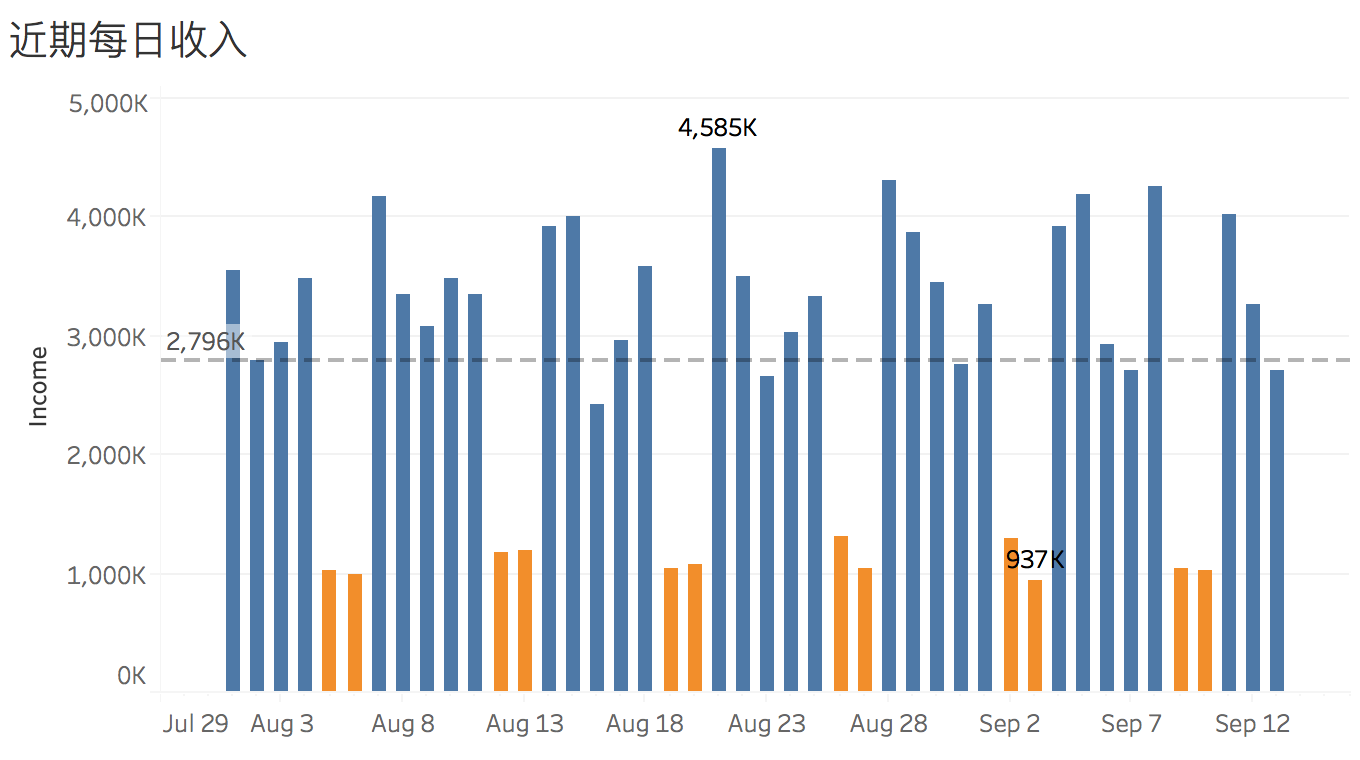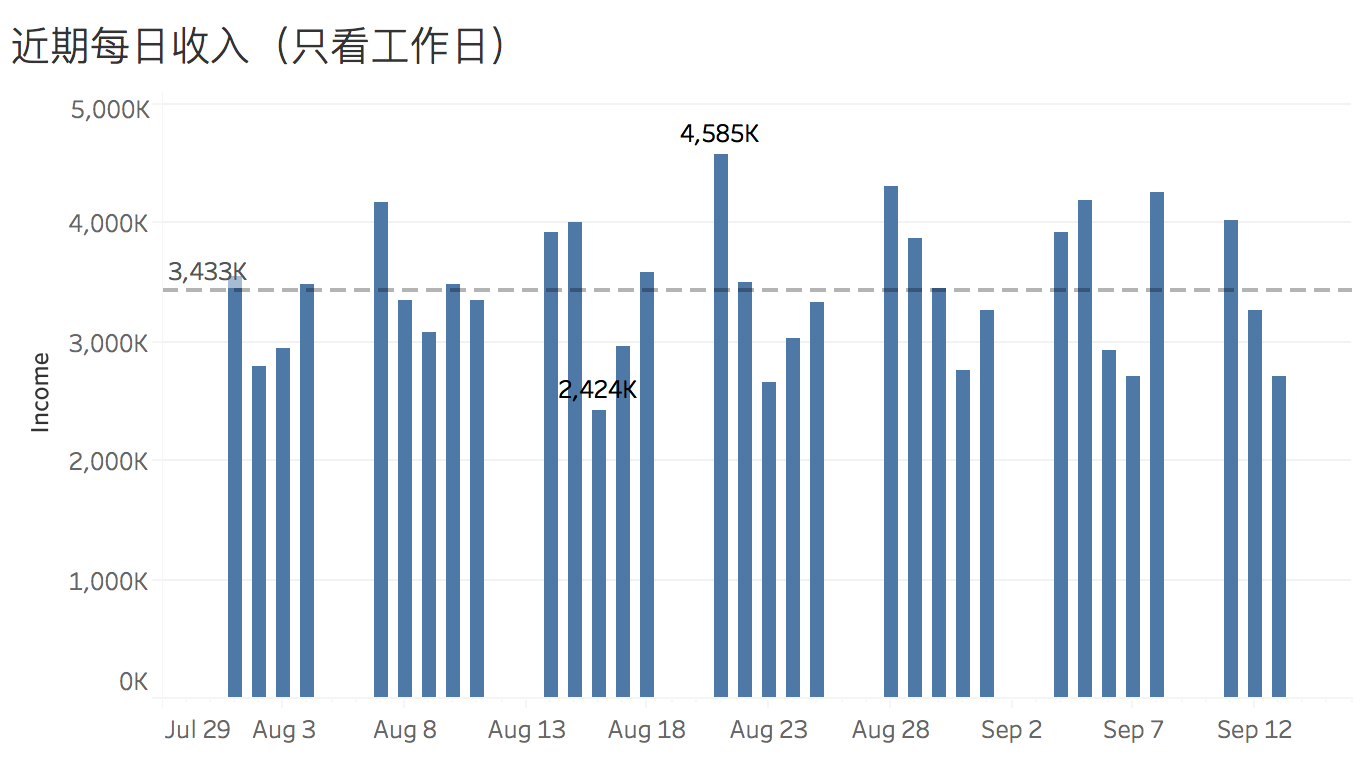``````字段名：weekday_income
if [is_weekend] then
null
else
[income]
end
``````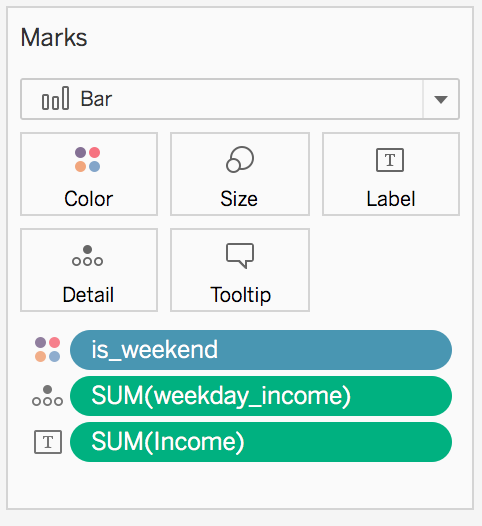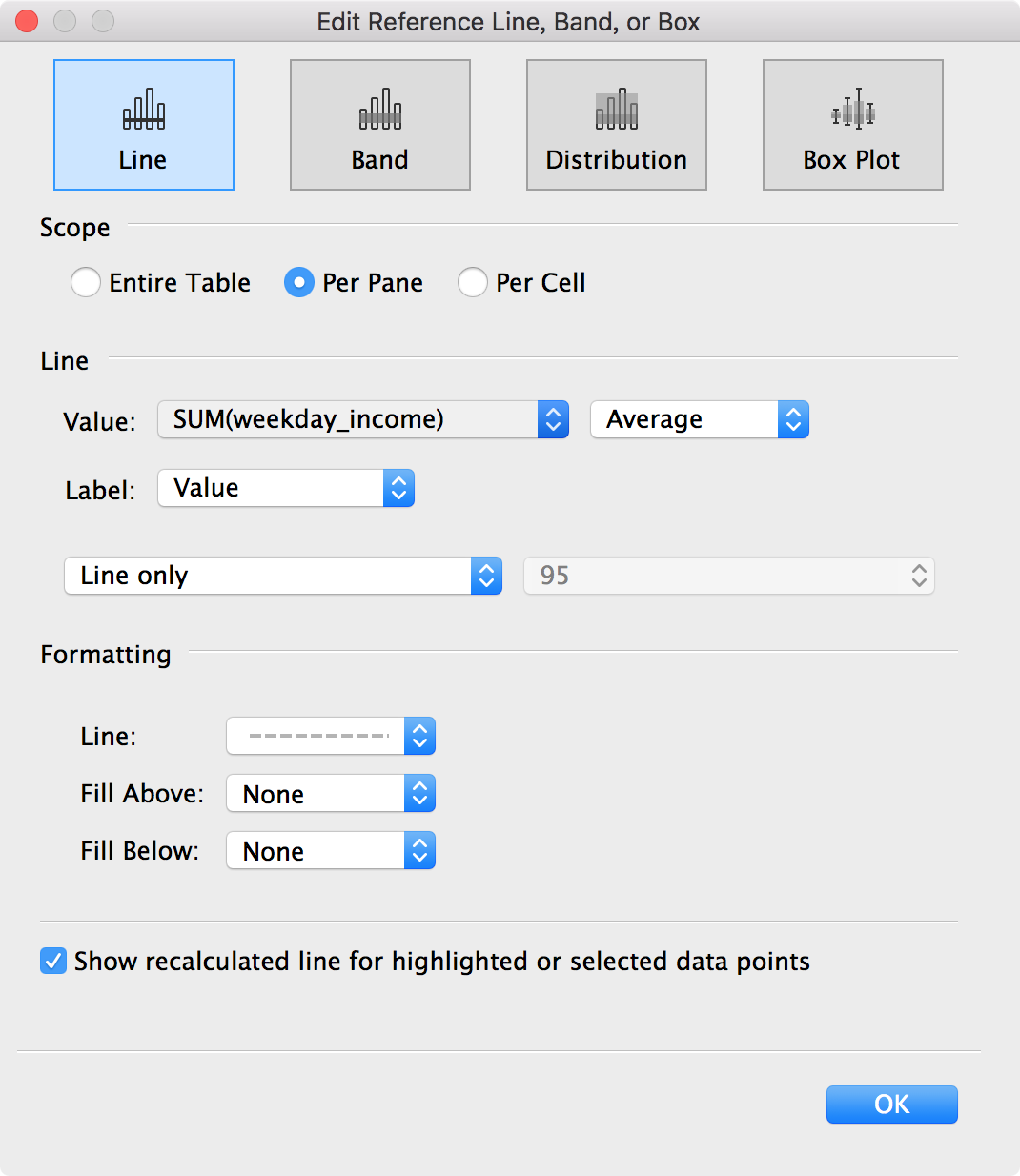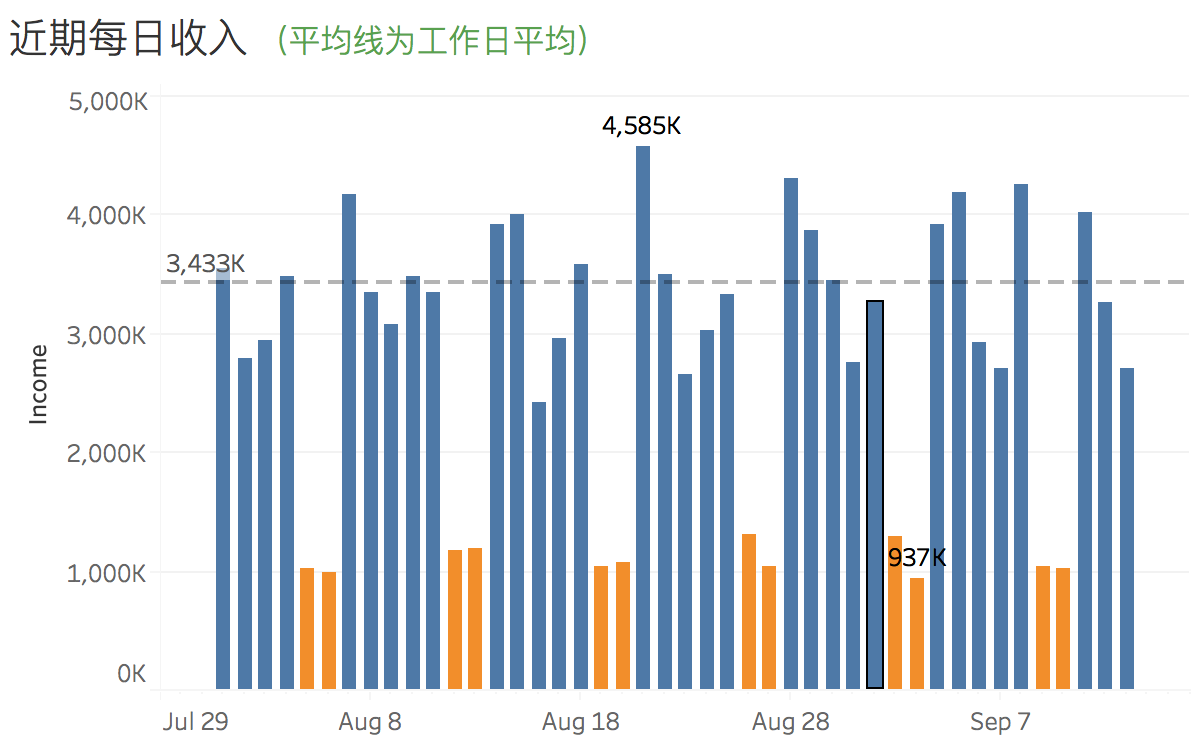``````TOTAL(AVG([weekday_income]))
``````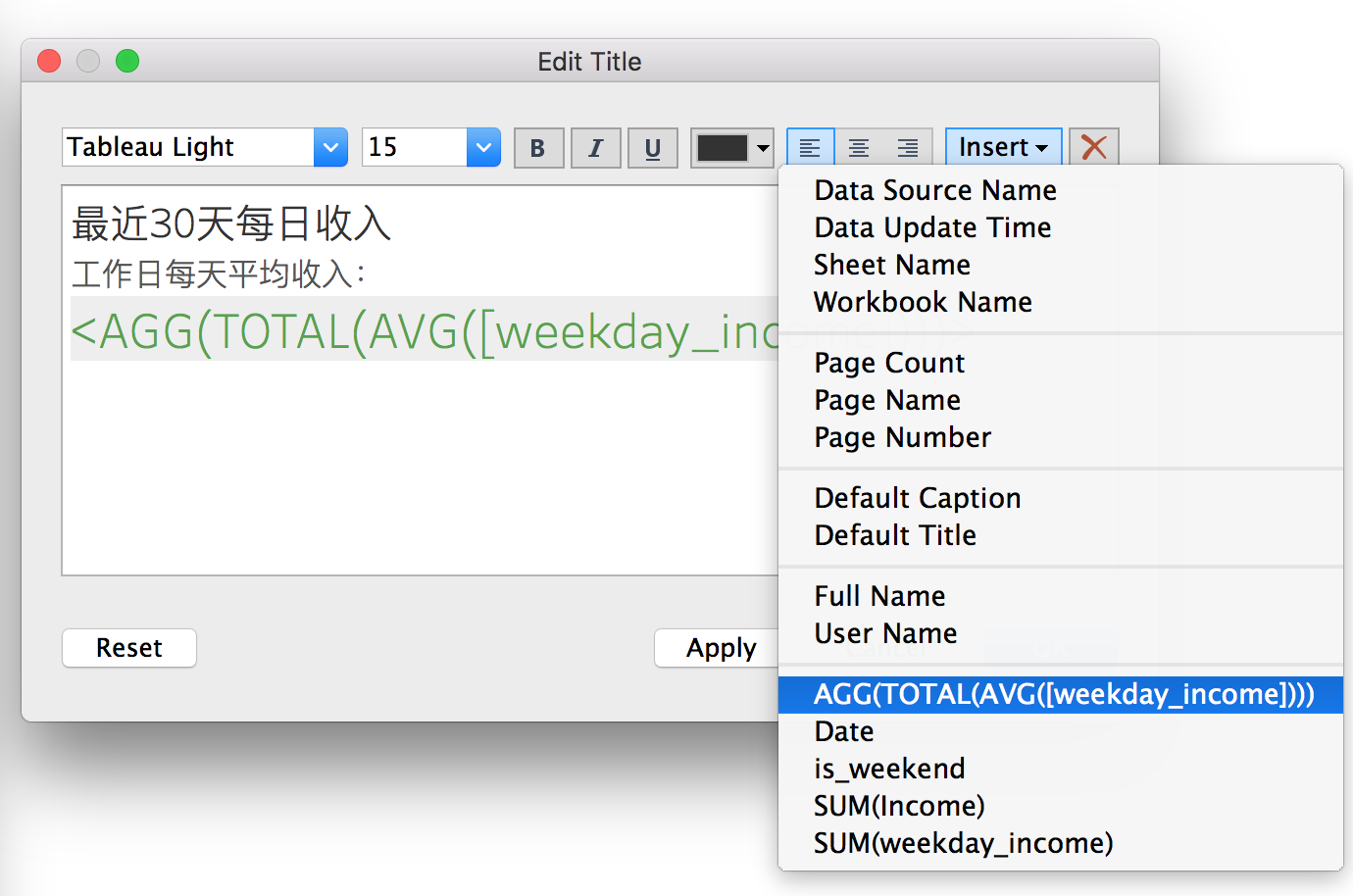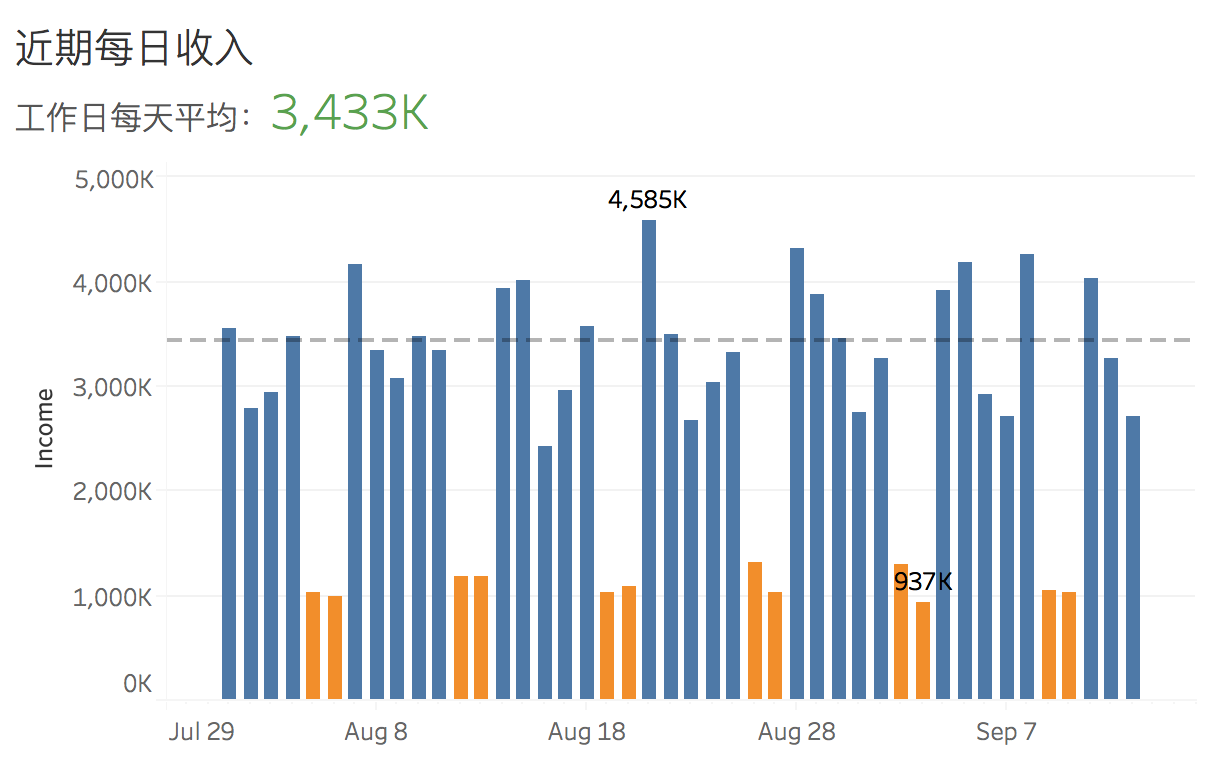[https://public.tableau.com/profile/jiyang#!/vizhome/workday_income/]

``````import datetime
import random

day_seed = [1.3, 1.2, 1, 1, 1.2, 0.4, 0.35]

d = datetime.date(2017, 6, 12)

print('date\tincome')
while d <= datetime.date.today():
d += datetime.timedelta(days=1)
day_index = d.weekday()
print( str(d) +
'\t' +
str(int(3000000 * random.uniform(0.8 * day_seed[day_index],
1.2 * day_seed[day_index]))))
``````